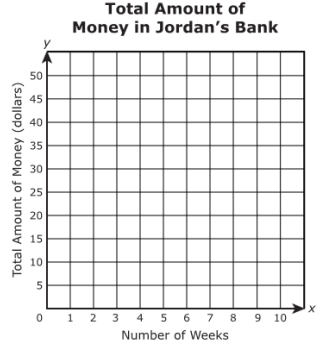# Patterns and the Coordinate Plane

## Objective

Solve real-world problems by graphing information given as a description of a situation in the coordinate plane and interpret coordinate values of points in the context of the situation.

## Common Core Standards

### Core Standards

?

• 5.G.A.2 — Represent real world and mathematical problems by graphing points in the first quadrant of the coordinate plane, and interpret coordinate values of points in the context of the situation.

• 5.OA.B.3 — Generate two numerical patterns using two given rules. Identify apparent relationships between corresponding terms. Form ordered pairs consisting of corresponding terms from the two patterns, and graph the ordered pairs on a coordinate plane. For example, given the rule "Add 3" and the starting number 0, and given the rule "Add 6" and the starting number 0, generate terms in the resulting sequences, and observe that the terms in one sequence are twice the corresponding terms in the other sequence. Explain informally why this is so.

?

• 4.OA.C.5

## Criteria for Success

?

1. Graph points represented in a description of a situation on a coordinate plane.

## Problem Set & Homework

### Discussion of Problem Set

• How did you solve #2? How did the graph make it easier to solve? What was difficult about the way the graph was set up, though?
• How did you solve #4? Did anyone create a table or draw a graph? Or did you use another strategy?
• What made #5 more challenging? How did you approach solving?
• How long would it take Dean to read 20 books in #5? How do you know? How many books could he read in 12 days? How do you know?

?

Jordan has $10 in the bank. Jordan earns$5 each week for doing chores, and he puts the money in the bank. After a certain number of weeks of doing chores, Jordan has $35. A graph is set up so that Jordan can record the total amount of money in the bank each week after putting in$5.a.   Which ordered pair represents the amount of money Jordan has in the bank before doing any chores?

1. a.   (0, 10)

2. b.   (0, 35)

3. c.   (10,0)

4. d.   (35,0)

b.   Which ordered pair represents the amount of money Jordan has after 4 weeks of doing chores?

1. a.   (4, 20)

2. b.   (4, 30)

3. c.   (20, 4)

4. d.   (30, 4)

c.   After how many weeks does Jordan have \$35? Show or explain your work.

#### References

PARCC Released Items Math Spring 2017 Grade 5 Released ItemsQuestion #25